• 1、常用的统计值相对应的python函数 平均数：np.mean() 总和：np.sum() 最大值：np.max() 最小值：np.min() 方差：np.var() 标准差：np.std() 频次（计数）: np.count() 中位数：np.median() 协方差： np.cov(x, y)...
1. 背景描述
数据分析中经常需要对数据特征的多种不同方式进行分组或归类，然后与统计函数结合使用，寻找信息的规律、数据间的差异或相关性等，从而更好地从数据中挖掘有价值的信息，服务于企业的运营和特定的问题解决。
本文主要在这两方面进行总结：

数据分析中常用的统计函数（共15个聚合函数-python）的用法
与4种不同的分组、归类方法结合使用的效果比较

2. 数据分析常用统计函数总结
2.1 常用的统计值对应的python函数

平均数：np.mean()
总和：np.sum()
中位数：np.median()
最大值：np.max()
最小值：np.min()
频次（计数）: np.size()
方差：np.var()
标准差：np.std()
乘积：np.prod()
协方差： np.cov(x, y)
偏度系数(Skewness): skew(x)
峰度系数(Kurtosis): kurt(x)
正态性检验结果: normaltest(np.array(x))
四分位数：np.quantile(q=[0.25, 0.5, 0.75], interpolation=“linear”)
四分位数：describe() – 显示25%, 50%, 75%位置上的数据
相关系数矩阵(Spearman/ Person/ Kendall)相关系数: x.corr(method=“person”))

2.2 示例代码
**基本使用方法 **
import numpy as np
import pandas as pd
import scipy.stats as ss

# 1. 常见统计函数的使用
x = [5, 7, 6, 5, 8, 4, 7, 6, 4, 5]
print("x的平均值:", np.mean(x))
print("x总和是:", np.sum(x))
print("x的中位数:", np.median(x))
print("x的最大值:", np.max(x))
print("x的最小值:", np.min(x))
print("x的数据个数:", np.size(x))
print("x的方差:", np.var(x))
print("x的标准差:", np.std(x).round(2))
print("x的乘积:", np.prod(x))
print("x的偏度(Skewness):", skew(x))
print("x的四分位数:", np.quantile(x, [0.25, 0.5, 0.75]))
print("x的正态检验结果:", ss.normaltest(np.array(x)))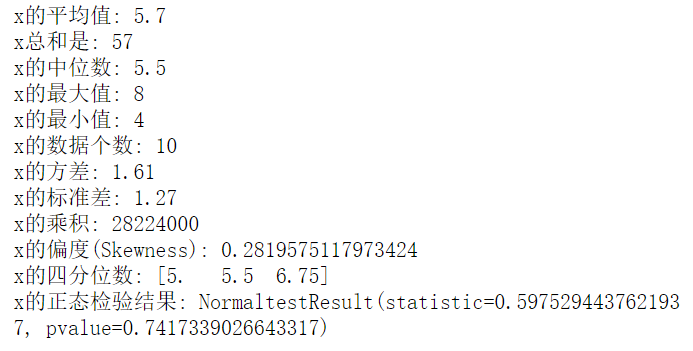# 2. 计算数据样本的相关系数矩阵：
x = pd.DataFrame([range(1, 5), range(2, 6)])
print("Pearson相关系数:\n", x.corr(method="pearson"))
print("Spearman相关系数\n:", x.corr(method="spearman"))
print("Kendall相关系数\n:", x.corr(method="kendall"))
x1 = x.loc
x2 = x.loc
print("x1与x2间的pearsom相关系数:", x1.corr(x2, method="pearson"))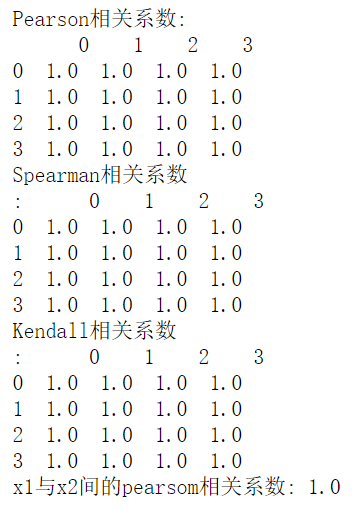3. 聚合函数与分组归类方法的联合使用（Python代码）
3.1 DataFrame数据表中分组进行统计 – 与groupby()联合使用
# 3.1.1 建立数据表
import numpy as np
import pandas as pd
df = pd.DataFrame({"名称": ["A", "B", "A", "A", "B", "A"],
"颜色": ["红色", "蓝色", "红色", "蓝色", "蓝色", "红色"],
"尺寸": ["大", "大", "小", "小", "大", "大"],
"厚度": [2, 5, 1, 2, 4, 5],
"数量": [10, 20, 15, 30, 10, 20]})
df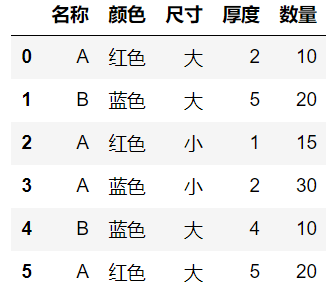# 3.1.2 使用groupby()根据“名称”对“数量”进行分组统计
df.groupby(["名称"])["数量"].sum()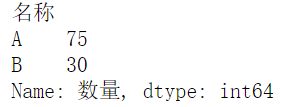# 3.1.3 groupby()函数传入分组列表，对数据进行多层分组统计
df.groupby(["名称", "颜色"])["数量"].sum()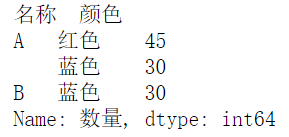# 3.1.4 在之前的基础上，对被统计的数据传入列表，达到多层分组分类统计的效果
df.groupby(["名称", "颜色"])[["数量", "厚度"]].sum()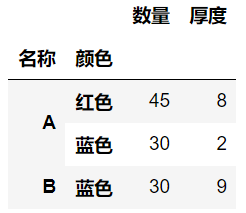3.2 数据表中多层筛选的应用 – 透视表pivot_table的应用
# 3.2.1 简单的分组分类统计汇总
pd.pivot_table(df, values=["数量"], index=["名称"], columns=["颜色"], aggfunc=np.sum,fill_value=0, margins=True)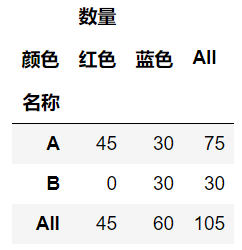# 3.2.2 多层级的分组和分类统计汇总
pd.pivot_table(df, values=["数量"], index=["名称", "尺寸"], columns=["颜色"], aggfunc=np.sum, fill_value=0, margins=True)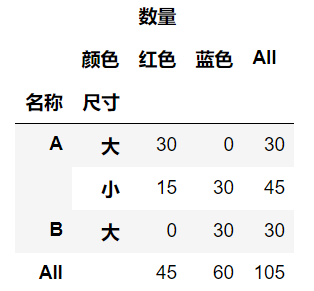# 3.2.3 多层分组统计不同指标的不同统计量
pd.pivot_table(df, values=["数量", "厚度"], index=["名称"], columns=["颜色"], aggfunc={"数量": np.sum, "厚度": np.var}, fill_value=0, margins=True)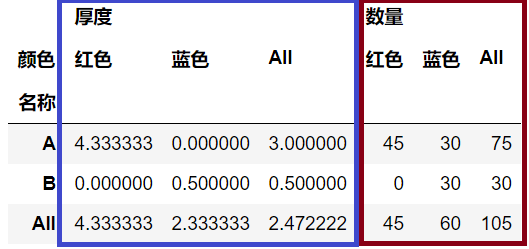蓝色框 – 分组计算厚度的方差
红色框 – 分组同步计算数量的总和
这种方法不但快速便捷，还能在同一个平面内展示不同指标使用不同的统计量计算的结果。
3.3 四分位数 – describe()的应用方法
# describe()函数 -- 对数据表中的所有的数值型进行快速的统计
df.describe()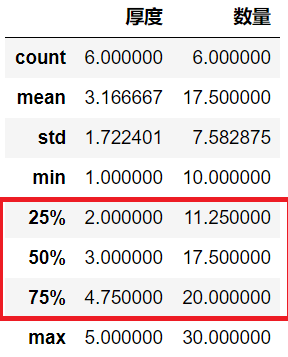3.4. 交叉表crossTab()函数
# 交叉表crossTab()函数 -- 交叉表是用于统计分组频率的特殊透视表
tab_ = pd.crosstab(df["名称"], df["颜色"], margins=True)
tab_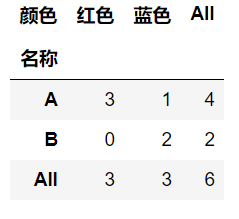3、结束语
综述以上4种方法，基本上都会认为pivot_table()相对而言是最便捷、灵活且功能强大的统计分析函数，也是在日常工作中常用的方法之一。其实，每种方法都有特色和针对的方向，根据实际情况灵活运用即可。


展开全文• Python count() 方法用于统计字符串里某个字符出现次数。可选参数为在字符串搜索开始与结束位置。 语法 str.count(sub, start= 0,end=len(string)) 参数 sub – 搜索子字符串 start – 字符串...
文章目录变量数学运算count()count()字符串count()list; count()tuplePrint 函数repr() 函数DEL删除函数POP 函数
变量数学运算
python数字运算 Python数字运算常用的有:加(+)、减(-)、乘(*)、除(/)、取模(%)、幂运算()**
其中除法运算符有两种，一种是真正的除法(/)保留小数,另一种是地板除(//)去除小数（地板除就是向下取整，去除小数部分）。
count()
count()字符串

str.count(sub, start= 0,end=len(string))

count()list; count()tuple

list.count(obj)
tuple.count(obj)

Print 函数
print() 方法的语法:

print(*objects, sep=’ ‘, end=’\n’, file=sys.stdout)

参数

objects – 复数，表示可以一次输出多个对象。输出多个对象时，需要用 , 分隔。
sep – 用来间隔多个对象，默认值是一个空格。
end – 用来设定以什么结尾。默认值是换行符 \n，我们可以换成其他字符串。
file – 要写入的文件对象。

返回值

无。

实例
print_demo = 'seosem'

print(print_demo, end= '/')
print(print_demo, end= '/')
print(print_demo, end= '/')


repr() 函数
repr() 函数将对象转化为供解释器读取的形式。
语法

以下是 repr() 方法的语法:
repr(object)

参数
object – 对象。

返回值
返回一个对象的 string 格式。

seo_title = ['奢侈品']
s= repr(seo_title)
print(s)


DEL删除函数
del()用于list列表操作，删除一个或者连续几个元素。
>>> a = [-1, 3, 'aa', 85] # 定义一个list
>>> a
[-1, 3, 'aa', 85]
>>> del a      # 删除第0个元素
>>> a
[3, 'aa', 85]
>>> del a[2:4]   # 删除从第2-3个元素。
>>> a
[3, 'aa']
>>> del a       # 删除整个list
>>> a
Traceback (most recent call last):
File "<stdin>", line 1, in <module>
NameError: name 'a' is not defined

numpy.delete()适用于numpy ndarray数组。但是numpy数组不支持删除数组元素，numpy.delete() 返回删除了某些元素的新数组。
import numpy as np

a = np.array([1, 2, 3, 4, 5, 6, 7, 8, 9])
index = [2, 3, 6]

new_a = np.delete(a, index)
print(new_a)        #Prints [1, 2, 5, 6, 8, 9]

del 也可以用于直接删除字符串，但不能删除字符串中具体的某个元素·

seo_title = '奢侈品'
del seo_title
>>>
TypeError: 'str' object doesn't support item deletion


unt 函数方法：

字符串
Python count() 方法用于统计字符串里某个字符出现的次数。可选参数为在字符串搜索的开始与结束位置。

语法
str.count(sub, start= 0,end=len(string))

参数
sub – 搜索的子字符串
start – 字符串开始搜索的位置。默认为第一个字符,第一个字符索引值为0。
end – 字符串中结束搜索的位置。字符中第一个字符的索引为 0。默认为字符串的最后一个位置。

返回值
该方法返回子字符串在字符串中出现的次数。

   实例：
chr= 'seosem'
print(chr.count('s'))
>>>
2


列表: list的count() 方法用于统计某个元素在列表中出现的次数。

count()方法语法：
list.count(obj)

参数
obj – 列表中统计的对象。

返回值
返回元素在列表中出现的次数。

实例

#以下实例展示了 count()函数的使用方法：

#!/usr/bin/python

aList = [123, 'xyz', 'zara', 'abc', 123];

print "Count for 123 : ", aList.count(123);
print "Count for zara : ", aList.count('zara');

以上实例输出结果如下：
>>>
Count for 123 :  2
Count for zara :  1

POP 函数

set.pop()是随机删除集合中的一个元素。

    set_this= {2,4,1,6,2}
set_this.pop()
print(set_this)



展开全文• DataFrame的函数df.head() 查看数据框df前几行数据df.tail() 查看数据框df最后几行数据df.info() 获取数据框df信息df.describe() 获取数据框df各项统计值df.index 查看数据框df行索引df.columns 查看数据...
DataFrame的函数df.head()	查看数据框df的前几行数据df.tail()	查看数据框df的最后几行数据df.info()	获取数据框df的信息df.describe()	获取数据框df的各项统计值df.index	查看数据框df的行索引df.columns	查看数据框df的列名df.shape	查看数据框df的形状，行数和列数Series数据的一些统计分析函数se.unique()	获取Series数据中的数值种类，一般用于分类数据，这里se为Series格式数据se.value_counts()	统计Series数据中的数值种类及其对应的数据个数se.mean()	计算Series数据的均值se.std()	计算Series数据的标准差se.median()	计算Series数据的中位数se.max()	计算Series数据的最大值se.min()	计算Series数据的最小值se.count()	计算Series数据的个数以上的统计数字都可以通过data.describe()函数得到所有属性的统计量。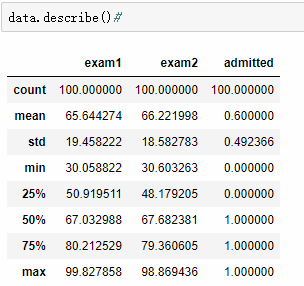count 是数据量。mean是平均值。std是标准差标准差（Standard Deviation） ，中文环境中又常称均方差，是离均差平方的算术平均数的平方根，用σ表示。标准差是方差的算术平方根。标准差能反映一个数据集的离散程度。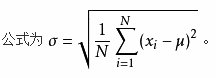min和 max分别代表的是最小值和最大值。剩下的25%，50%和75%，将数据按大小排列后，分别在总数据中25%，50%和75%位置的数据。
展开全文• 内置函数(无需导入) print：打印，输出 input：输入 abs：求绝对值 type：获取变量类型 chr：将ASCII数值转换为字符 ord：将字符转换为对应ASCII数值 id：获取变量地址 len：统计元素个数 range：产生...
欢迎错误指正和留言讨论哦

内置函数(无需导入)

print：打印，输出

input：输入

abs：求绝对值

type：获取变量的类型

chr：将ASCII数值转换为字符

ord：将字符转换为对应的ASCII数值

id：获取变量地址

len：统计元素个数

range：产生连续整数的可迭代对象

enumerate：枚举可迭代对象

强制类型转换：

int

float

str

list

tuple

set

dict

max：求多个参数的最大值，或可迭代对象中的最大元素

min：最小值

sum：求和，可迭代对象元素求和

pow：求幂，pow(2, 3)等价于2 ** 3

round：四舍五入，可以指定保留位数

hex：16进制

oct：8进制

bin：2进制


展开全文• python中，主要两个库用于数据探索，一个是Pandas（用于数据分析，这可能是数据分析领域最强的python库），另一个是Matplotlib（用于数据可视化，绘制图表，起源于MATLAB）。 1.基本统计特征函数 下面介绍的函数...数据探索
• 目录一、abs 函数--获取数字绝对值二、divmod 函数--获取两个数值商和余数三、len 函数--获取对象长度或项目个数四、max 函数--获取可迭代对象(或元素)最大值五、min 函数--获取可迭代对象(或元素)最小值...
• 统计一篇文章中，所有词语和标点符号个数，使用下面方法。len函数对于重复词语，会进行多次计数 len(text3) 2，获取文章中全部单词 获取文章中全部单词使用函数set，它会输出text3中全部单词，...
• Python常用命令 # 1. 显示所有列 pd.set_option('display.max_columns', None) # 2. 显示所有行 pd.set_option('display.max_rows', None) # 3. 设置value显示长度为100，默认为50 pd.set_option('max_colwidth',...
• 1、cmp(list1, list2)：比较两个列表元素 (python3已丢弃) 2、len(list)：列表元素个数 3、max(list)：返回列表元素最大值 4、min(list)：返回列表元素最小值 5、list(seq)：将元组转换为列表 2 列表操作常用...列表
• 1.整数转二进制 正数 get = bin(n).replace('0b', '') 负数 用补码表示 get = bin(n & 0xffffffff).replace('0b','') 2.an次方 pow(a, n) ...3.统计数组中各元素出现次数 ...map()会根据提供的函数对指定...
• 1.os.path.isfile(): 用于判断某一对象（绝对路径）是否为目录 https://www.jianshu.com/p/582910d13501 2.Python读取系统文件夹内所有文件并统计数量方法 https://www.jb51.net/article/149404.htm ...
• 数组中常用的统计函数 假设有一个二维数组t t = np.arange(24).reshape((4,6)) print(t) # [[ 0 1 2 3 4 5] # [ 6 7 8 9 10 11] # [12 13 14 15 16 17] # [18 19 20 21 22 23]] axis属性：指定统计的轴方向 axis=0 ...numpy 数据分析
• 了解详情可见：Python中的数据可视化工具与方法——常用的数据分析包numpy、pandas、statistics的理解实现和可视化工具matplotlib的使用 pandas中提供了大量统计特征函数统计绘图函数，因绘图函数依赖于matplotlib...数据挖掘 数据分析
• 1、如何统计一篇文章中出现频率最高 5 个单词? import re d={} str='If we want to" run Locust \ / distributed on multiple machines we would also have to specify the master host when starting the slaves ......

# python常用的统计函数python 订阅